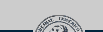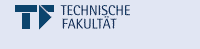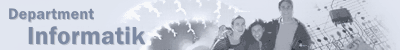# Pattern Recognition [PR]

Summary
This lecture gives an introduction into the basic and commonly used classification concepts. First the necessary statistical concepts are revised and the Bayes classifier introduced. Further concepts include generative and discriminative models like logistic regression, the Gaussian classifier, Linear Discriminant Analysis, the Perceptron and Support Vector Machines (SVMs). Finally more complex methods like the Expectation Maximization Algorithm and Hidden Markov Models are discussed. In addition to the mentioned classifiers, methods necessary for practical application like dimensionality reduction, optimization methods and the use of kernel functions are explained. In the tutorials the methods and procedures which are presented in this lecture are illustrated using theoretical and practical exercises.

Dates & Rooms:
Wednesday, 13:30 - 14:30; Room: H10
Monday, 12:00 - 14:00; Room: H10

Lecturer
Hahn, Dieter